# BALM: Bundle Adjustment for Lidar Mapping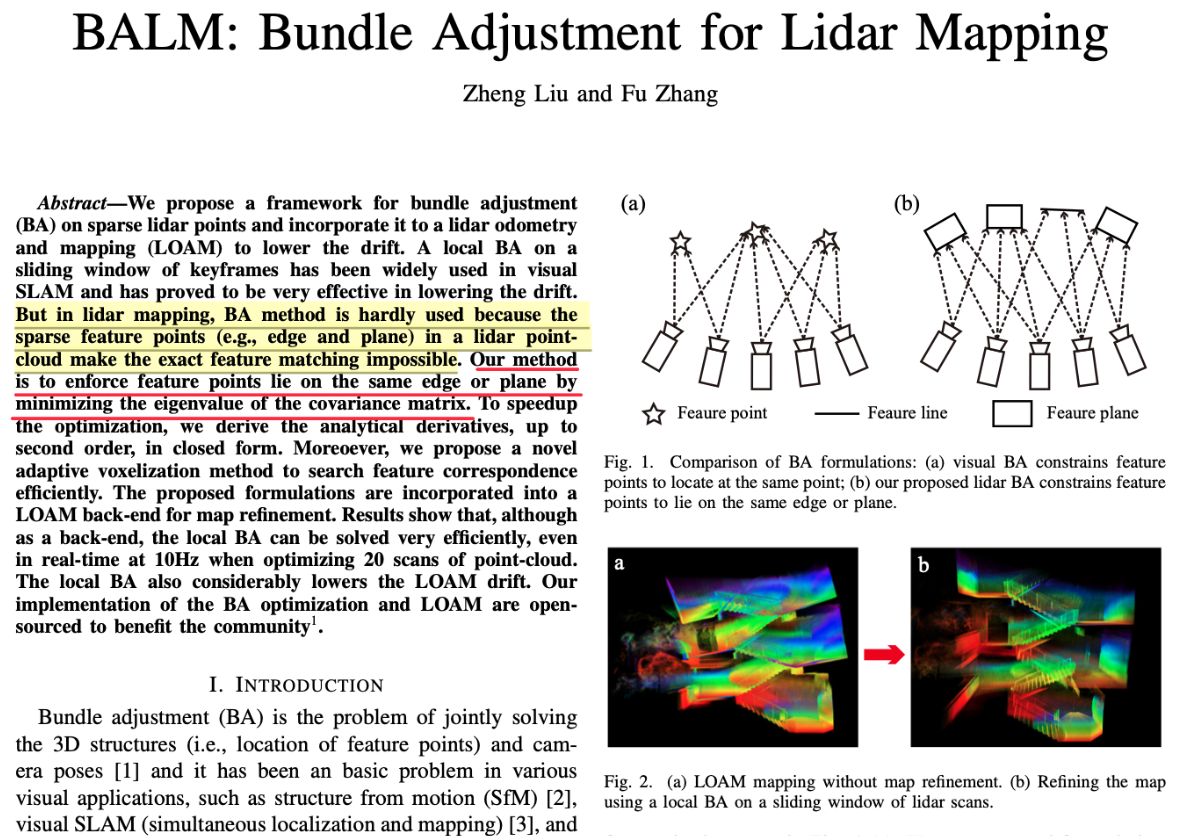# 介绍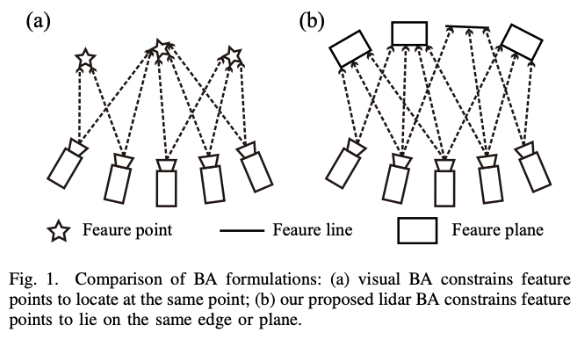• 提出了激光雷达BA框架，不像视觉ba那样，而是通过最小化协方差矩阵来约束特征点到边缘线或者平面的距离，还推到了cost func(如特征值)对激光雷达位姿的梯度和Hessian矩阵
• 提出了自适应体素化方法来快速搜索特征关联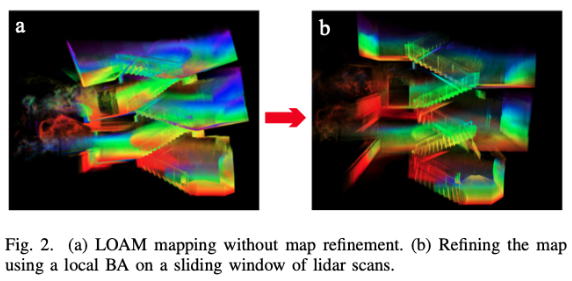# BA推导

## 问题描述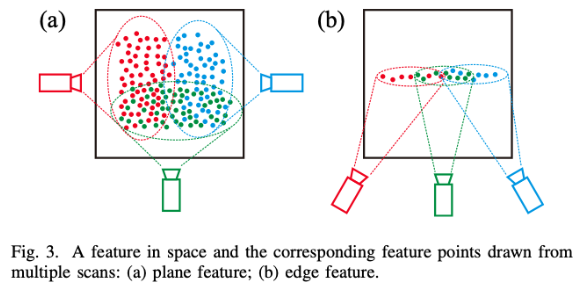• $$i\in \{1,\cdots,N\}$$
• $$s_i \in \{1,\cdots,M\}$$
• M帧的位姿记为$$T=(T_1,\cdots,T_M)$$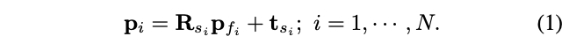### 对于平面特征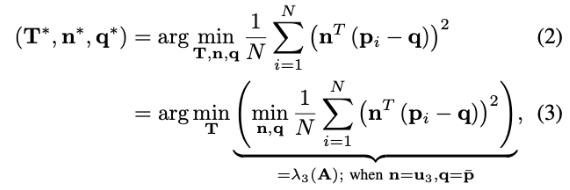• $$p_i$$：根据待估计位姿投影到全局地图上的特征点
• $$q$$：某个特征上的点（边缘线内、平面内）
• $$\vec{n}$$: 边缘线的方向向量或者是平面法向量
• 因此，上式即为最小化投影点到特征的距离

$\arg \min _{\mathbf{T}, \mathbf{n}, \mathbf{q}} \mathbf{n}^T\left(\min _{\mathbf{q}} \frac{1}{N} \sum_{i=1}^N\left(\mathbf{p}_i-\mathbf{q}\right)\left(\mathbf{p}_i-\mathbf{q}\right)^T\right) \mathbf{n}$

$\mathbf{q}^*=\frac{1}{N} \sum_{i=1}^N \mathbf{p}_i$

（实际上，$$\mathbf{q}^*$$是平面上的点都能满足）

$\mathbf{A} = \frac{1}{N} \sum_{i=1}^N\left(\mathbf{p}_i-\mathbf{q}^*\right)\left(\mathbf{p}_i-\mathbf{q}^*\right)^T$

$\arg \min _{\mathbf{T}, \mathbf{n}} \mathbf{n}^T \mathbf{A n}$

$\lambda_{\min }(\mathbf{M}) \leq \frac{\mathbf{x}^T \mathbf{M x}}{\mathbf{x}^T \mathbf{x}} \leq \lambda_{\max }(\mathbf{M}), \forall \mathbf{x} \neq \mathbf{0}$

$\mathbf{T}^*=\arg \min _{\mathbf{T}} \lambda_{\min }(\mathbf{A})$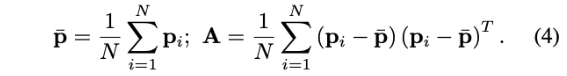### 对于边缘线特征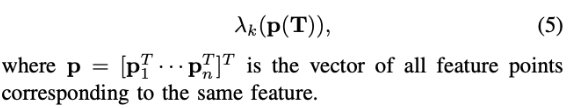## 微分

### 定理一### 定理二

• $$i \neq k$$时, $$\lambda_i \neq \lambda_k$$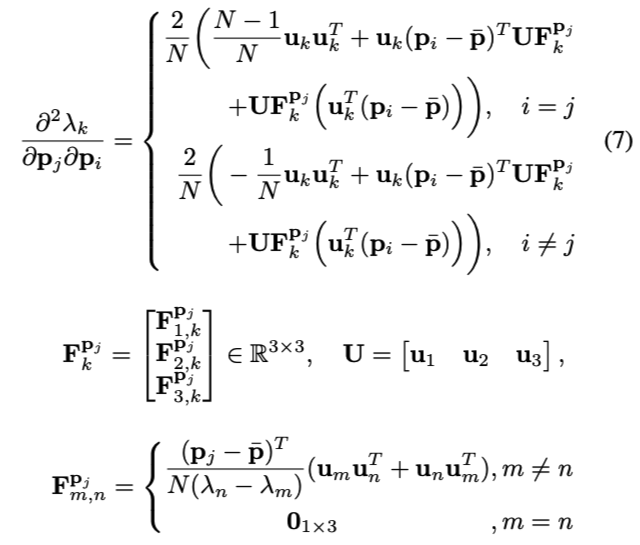## 二阶微分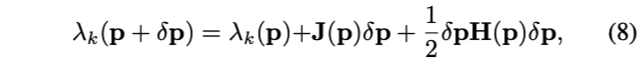• $$J(p)$$ 是雅可比矩阵，第i个元素按式(6)计算
• $$H(p)$$ 是Hessian矩阵，其中第i行第j列元素按式(7)计算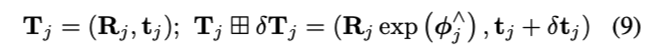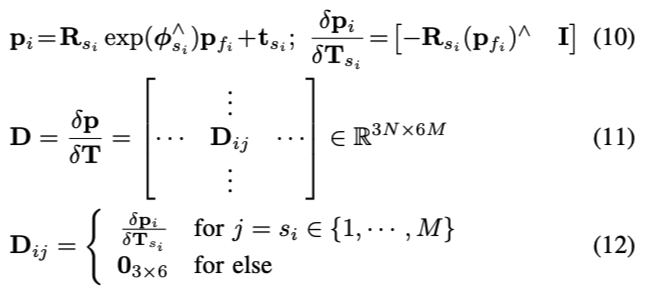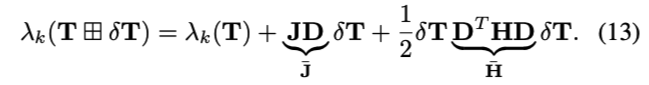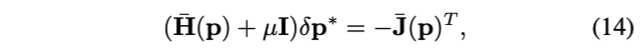# 附录A

## 证明：定理一

• $$\boldsymbol{\Lambda}$$是对角化后的矩阵

• $$\mathbf{u}_k^T\left(\mathbf{p}_j-\overline{\mathbf{p}}\right)\left(\mathbf{p}_j-\overline{\mathbf{p}}\right)^T \mathbf{u}_k$$ = $$\left(\mathbf{p}_j-\overline{\mathbf{p}}\right)^T \mathbf{u}_k \mathbf{u}_k^T\left(\mathbf{p}_j-\overline{\mathbf{p}}\right)$$
• 可以看做是关于点$$p_i$$的函数$$\mathbf{B}(p_i) = \mathbf{P}^{T}(p_i) \mathbf{P}(p_i)$$，其中$$\mathbf{P}(p_i) = \mathbf{u}_k^T\left(\mathbf{p}_j-\overline{\mathbf{p}}\right)$$
• 那么，可以将上面的求导看作是链式求导：

\begin{aligned} \frac{\partial \lambda_k}{\partial \mathbf{p}_i} &=\frac{1}{N} \sum_{j=1}^N \frac{\partial \mathbf{B}(p_i)}{\partial p_i} \\ &=\frac{1}{N} \sum_{j=1}^N \frac{\partial \mathbf{P}^{T}(p_i) \mathbf{P}(p_i)}{\partial p_i} \\ &=\frac{1}{N} \sum_{j=1}^N 2 (\mathbf{P}^{T}(p_i))_{const} \frac{\partial \mathbf{P}(p_i)}{\partial p_i} \\ \end{aligned}

• $$\overline{\mathbf{p}} = \frac{1}{N} \mathbf{p}_i$$

## 证明：定理二$$\mathbf{C}^q$$的对角线元素是零，与式（18）相似，使用q来替换p，有：• $$\mathbf{e}_k$$是3x1的向量，并且第k个元素是1，其他是0
• 特征向量$$\mathbf{u}_m$$ and $$\mathbf{u}_n$$被视为恒定向量\begin{aligned} \frac{\partial \mathbf{u}_k}{\partial \mathbf{p}_j}&=\left[\begin{array}{lll} \frac{\partial \mathbf{U} \mathbf{e}_k}{\partial x_j} & \frac{\partial \mathbf{U} \mathbf{e}_k}{\partial y_j} & \frac{\partial \mathbf{U} \mathbf{e}_k}{\partial z_j} \end{array}\right] \\ &= \mathbf{U}\left[\begin{array}{lll} \mathbf{C}_{1, k}^{x_j} & \mathbf{C}_{1, k}^{y_j} & \mathbf{C}_{1, k}^{z_j} \\ \mathbf{C}_{2, k}^{x_j} & \mathbf{C}_{2, k}^{y_j} & \mathbf{C}_{2, k}^{z_j} \\ \mathbf{C}_{3, k}^{x_j} & \mathbf{C}_{3, k}^{y_j} & \mathbf{C}_{3, k}^{z_j} \end{array}\right]\\ &= \mathbf{U} \left[\begin{array}{l} \mathbf{F}_{1, k}^{\mathbf{p}_j} \\ \mathbf{F}_{2, k}^{\mathbf{p}_j} \\ \mathbf{F}_{3, k}^{\mathbf{P}_j} \end{array}\right] \end{aligned}\begin{aligned} \mathbf{F}_{m, n}^{\mathbf{p}_j} &=\left[\begin{array}{lll} \mathbf{C}_{m, n}^{x_j} & \mathbf{C}_{m, n}^{y_j} & \mathbf{C}_{m, n}^{z_j} \end{array}\right] \in \mathbb{R}^{1 \times 3} \\ &=\left\{\begin{array}{cc} \frac{1}{\lambda_n-\lambda_m} \frac{\partial \mathbf{u}_m^T \mathbf{A} \mathbf{u}_n}{\partial \mathbf{p}_j}, & m \neq n \\ \mathbf{0} & , m=n \end{array}\right. \end{aligned}（因为$$\frac{\partial \lambda_k}{\partial \mathbf{p}_i}$$是 1x3 矩阵，但是Hessian是3x3矩阵，所以下面要对$$\frac{\partial \lambda_k}{\partial \mathbf{p}_i}$$的结果进行转秩，使得 3x1向量 对 1x3向量求导，才能得到3x3矩阵）

\begin{aligned} \frac{\partial}{\partial \mathbf{p}_j}\left(\frac{\partial \lambda_k}{\partial \mathbf{p}_i}\right)&= \frac{2}{N} \frac{\partial \left ( \left(\mathbf{p}_i-\overline{\mathbf{p}}\right)^T \mathbf{u}_k \mathbf{u}_k^T \right)^{T}}{\partial \mathbf{p}_j} \\ &= \frac{2}{N} \frac{\partial \mathbf{u}_k \mathbf{u}_k^T \left(\mathbf{p}_i-\overline{\mathbf{p}}\right) }{\partial \mathbf{p}_j} \\ &= \frac{2}{N} \frac{\partial \mathbf{u}_k \left ( \mathbf{u}_k^T \left(\mathbf{p}_i-\overline{\mathbf{p}}\right) \right ) }{\partial \mathbf{p}_j} \\ &= \frac{2}{N} \left( \frac{\partial \mathbf{u}_k }{ \partial \mathbf{p}_j } \left ( \mathbf{u}_k^T\left(\mathbf{p}_i-\overline{\mathbf{p}}\right) \right ) + \mathbf{u}_k \frac{\partial \left ( \mathbf{u}_k^T\left(\mathbf{p}_i-\overline{\mathbf{p}}\right) \right )}{\partial \mathbf{p}_j} \right) \\ &= \frac{2}{N} \left( \frac{\partial \mathbf{u}_k }{ \partial \mathbf{p}_j } \left ( \mathbf{u}_k^T\left(\mathbf{p}_i-\overline{\mathbf{p}}\right) \right ) + \mathbf{u}_k \mathbf{u}_k^T \frac{\partial \left(\mathbf{p}_i-\overline{\mathbf{p}}\right)}{\partial \mathbf{p}_j} + \mathbf{u}_k \left(\mathbf{p}_i-\overline{\mathbf{p}}\right)^{T} \frac{\partial \mathbf{u}_k }{ \partial \mathbf{p}_j } \right) \end{aligned}